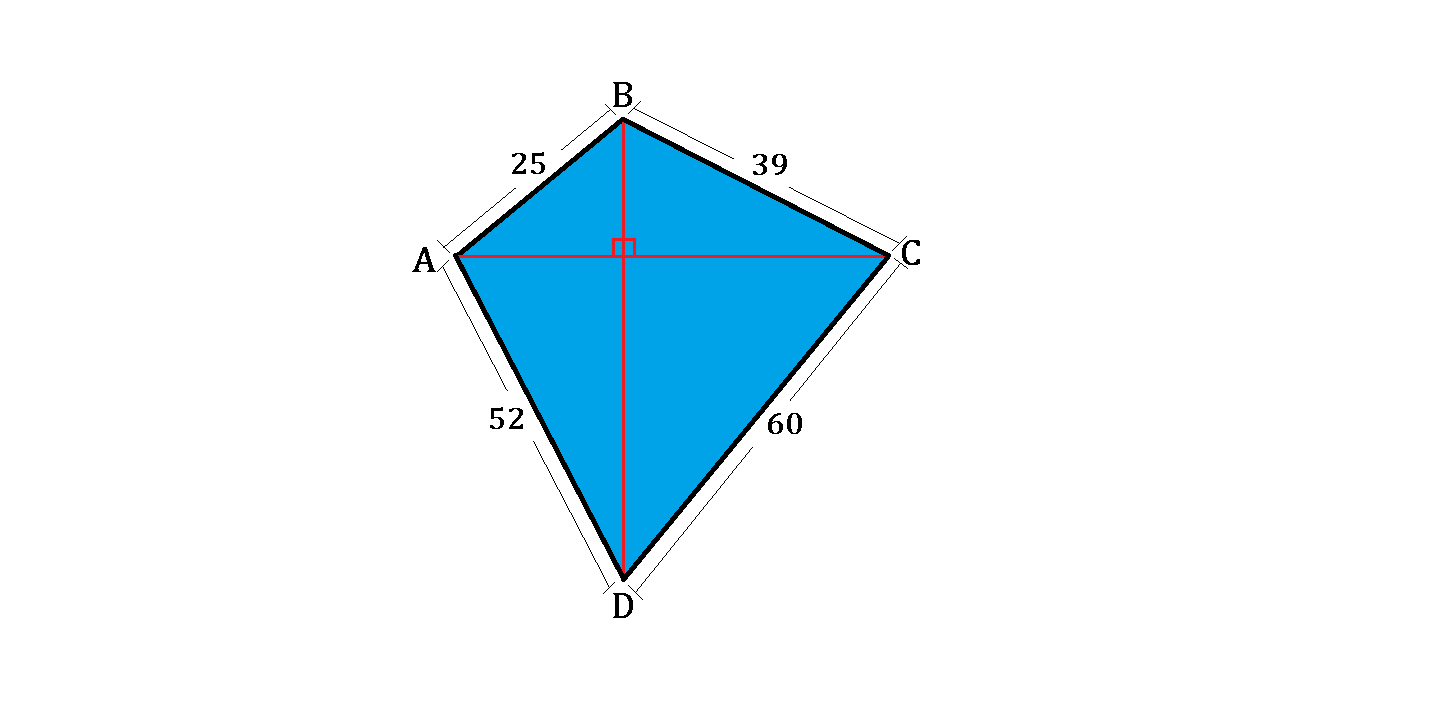# Four Right Triangles in OneLet $ABCD$ be a quadrilateral with $AB = 25, BC = 39, CD = 60$, and $DA = 52$, as shown above.

If the two diagonals are perpendicular to each other, yielding 4 Pythagorean triples for the 4 right triangles, and $AC : BD = 8 : 9$, what is the area of quadrilateral $ABCD?$

×